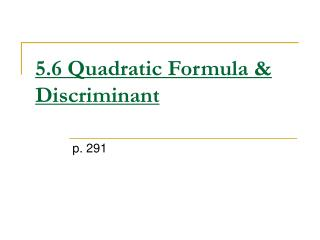DownloadDownload Presentation# 5.6 Quadratic Formula & Discriminant

Download Presentation## 5.6 Quadratic Formula & Discriminant

- - - - - - - - - - - - - - - - - - - - - - - - - - - E N D - - - - - - - - - - - - - - - - - - - - - - - - - - -
##### Presentation Transcript

1. Discriminant: b2-4ac • The discriminant tells you how many solutions and what type you will have. • If the discrim: Is positive – 2 real solutions Is negative – 2 imaginary solutions Is zero – 1 real solution

2. Find the discriminant and give the number and type of solutions. 9x2+6x+1=0 a=9, b=6, c=1 b2-4ac=(6)2-4(9)(1) =36-36=0 1 real solution 9x2+6x-4=0 a=9, b=6, c=-4 b2-4ac=(6)2-4(9)(-4) =36+144=180 2 real solutions c. 9x2+6x+5=0 a=9, b=6, c=5 b2-4ac=(6)2-4(9)(5) =36-180=-144 2 imaginary solutions Examples

3. Quadratic Formula(Yes, it’s the one with the song!) If you take a quadratic equation in standard form (ax2+bx+c=0), and you complete the square, you will get the quadratic formula!

4. When to use the Quadratic Formula Use the quadratic formula when you can’t factor to solve a quadratic equation. (or when you’re stuck on how to factor the equation.)

5. Examples • 3x2+8x=35 3x2+8x-35=0 a=3, b=8, c= -35 OR

6. -2x2=-2x+3 -2x2+2x-3=0 a=-2, b=2, c= -3

7. Assignment